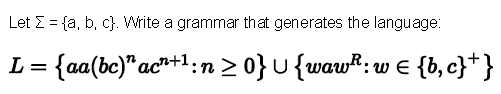# Answered! Let sigma = {a, b, c}. Write a grammar that generates the language: L = {aa (bc)^n ac^n + 1: n greater than or equal…Let sigma = {a, b, c}. Write a grammar that generates the language: L = {aa (bc)^n ac^n + 1: n greater than or equal to 0} Union {waw^R: w elementof {b, c}^+}